## 《结构力学基础》在线平时作业1-00001

《结构力学基础》在线平时作业1-00001

------------------------
1.等截面直梁如图所示，如果截面面积不变，以下哪种截面形状梁的强度最高（   ）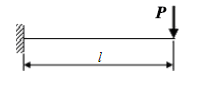A.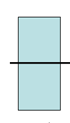B.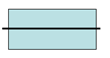C.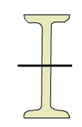D.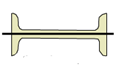2.以下说法错误的是（  ）

A.梁的轴线在弯曲变形后仍然与横截面垂直

B.梁的转角等于挠曲线的倾角

C.挠曲线方程的一阶导数等于转角方程

D.受纯弯的等截面直梁，梁弯曲后的曲率半径与弯矩成正比

3.下图所示平衡平面汇交力系，已知F1=10kN，则（  ）是正确的。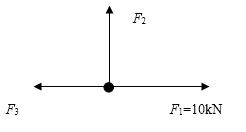A.

F2=0  ,    F3=10kN

B.

F2=10kN ,   F3=10kN

C.

F2=10kN ,   F3=0

D.

F2=0 ,     F3=0

4.矩形截面梁当横截面的高度增加一倍，宽度减小一半时，则刚度变化为（
A.

B.

C.

D.

5.下图所示杆件，B为中点，（   ）是平衡力系
A.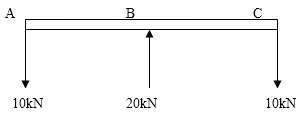B.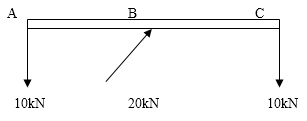C.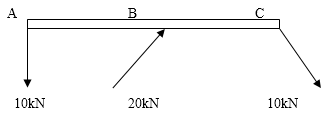D.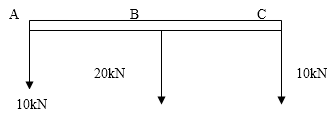6.杆件在下面哪种约束下被约束处不能有转角发生（   ）
A.

B.

C.

D.

7.变截面圆杆，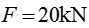AB段面积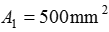BC段面积，两段长度均为500mm，E=200GPa, 则杆件的总变形量为（   ）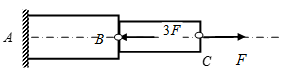A.

0.125mm

B.

-0.075mm

C.

-0.2mm

D.

0.325mm

8.下图所示结构（   ）是固定端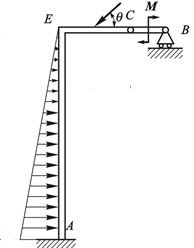A.

A

B.

B

C.

C

D.

E

9.图示结构，AB杆件B处的剪力为（   ）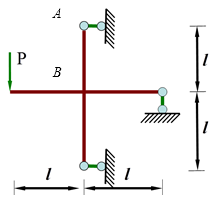A.

2P

B.

0

C.

-P

D.

P

10.AB=1m，q=100 kN/m，则B点的约束反力（    ）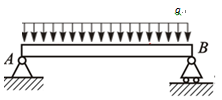A.

50kN

B.

100kN

C.

200 kN

D.

10kN

11.下面物体的整体受力图哪个是正确的（ )

A.B.C.D.12.下图所示的复刚结点相当于几个单刚结点（   ）A.1
B.2
C.3
D.4

13.下面描述做不正确的是（  ）

A.固定端也称作插入端
B.光滑面约束的约束反力方向一定与接触面垂直。
C.没有主动力作用的构件一定是二力杆
D.柔性体约束不能给被约束物体压力作用

14.下图所示长为l梁的边界条件为(     )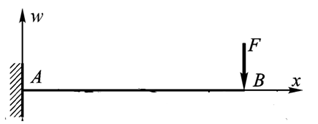A.

x=0, w=0; x=0, w’=0

B.

x=l, w=0; x=l, w’=0

C.

x=0, w=0; x=l, w=0

D.

x=0, w’=0; x=l, w=0

15.下图所示结构，AB=1m， F1=10 kN，作用于AB 的中点，F2=5 kN ，M=5 kNm， BC=0.5m，则A处的约束反力（  ）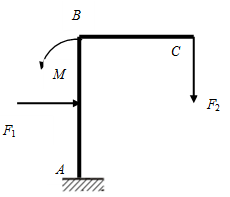A.

B.

C.

D.

------------------------
1.当力矩的中心在力线上时,力矩为零
T.对
F.错

2.力对点之矩顺时针为正
A.正确
B.错误

3.等截面直杆件轴向拉压变形时,轴向变形量与材料有关,弹性模量越大,变形越小
T.对
F.错

4.活动铰支座给被约束物体的约束反力总是垂直于支撑面指向被约束物体
A.正确
B.错误

5.刚架中如果包含铰接点，铰接点处的弯矩值一定为零
A.正确
B.错误

6.三个刚片两两通过单铰相连,必然会组成无多余约束的几何不变体系
T.对
F.错

7.力偶可以进一步简化为一个力
T.对
F.错

8.下图所示表示的是同样作用效果的平面力偶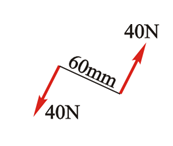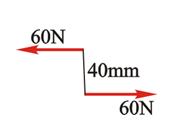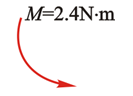A.正确
B.错误

9.平衡的平面一般力系中各力在任意坐标轴上投影代数和为零
T.对
F.错

10.截面和长度相同的两根杆，受相同的轴向拉力作用发生轴向拉压变形，一根为铁，一根为钢，两根杆的变形相同
A.正确
B.错误

11.平面一般力系中不能包含力偶
A.正确
B.错误

12.下图所示的力对O点之矩等于零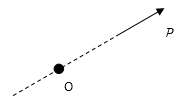A.正确
B.错误

13.不管由多少个力构成的平面汇交力系,简化结果都是一个作用在会交点的合力
T.对
F.错

14.刚架上有几种力偶作用处左右截面,弯矩图有突变
T.对
F.错

15.瞬变体系是指在发生微小的位移后即转化为几何不变的体系
T.对
F.错

16.联结两个刚片的两根平行连杆相当于一个连杆的作用
T.对
F.错

17.力在坐标轴上的投影一定为正值
A.正确
B.错误

18.下图所示表示的是同样作用效果的平面力偶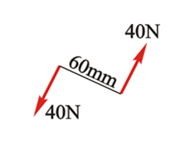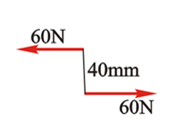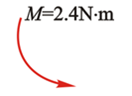A.正确
B.错误

19.在几何组成分析可以通过计算结构的来完成
A.正确
B.错误

20.扭转变形的变形特点是横截面的相对转动
T.对
F.错